Anzeige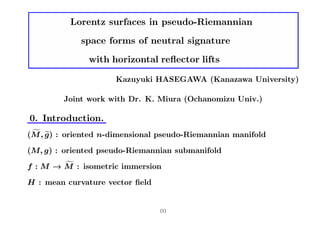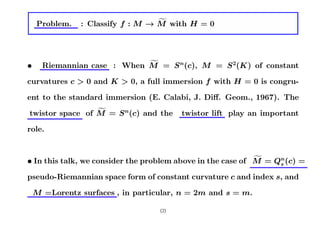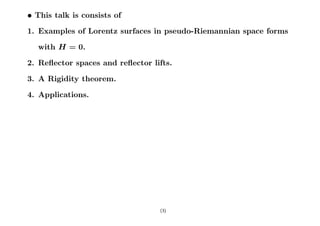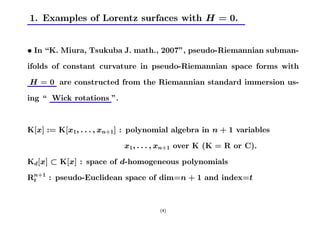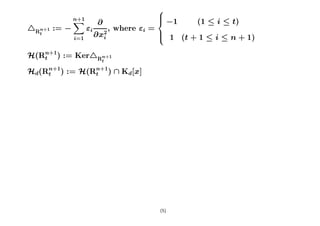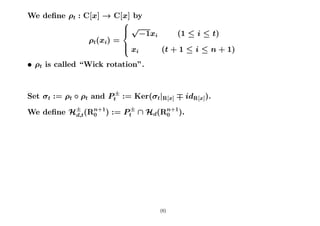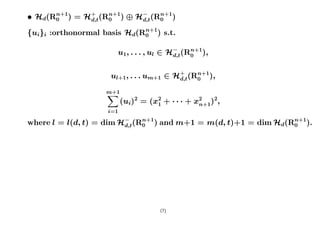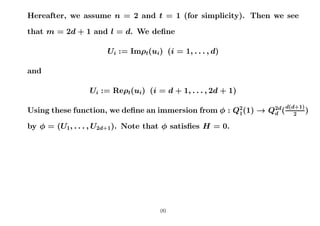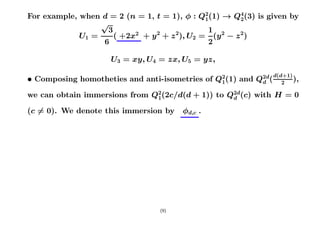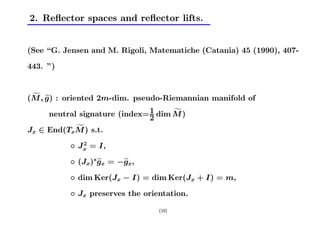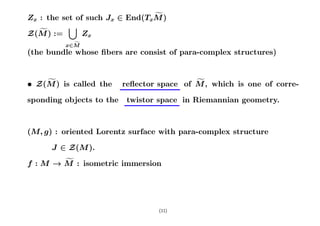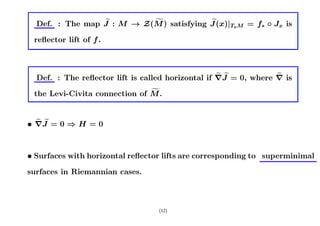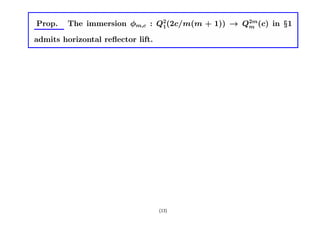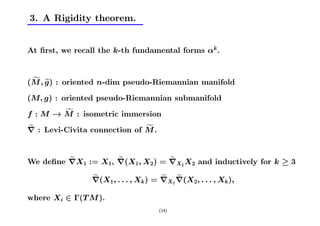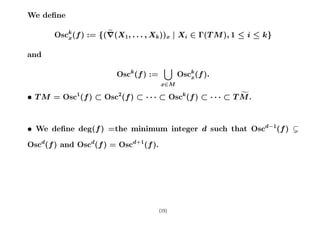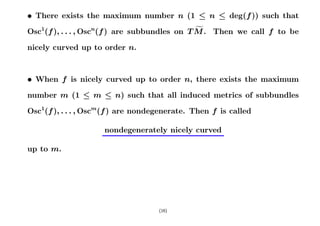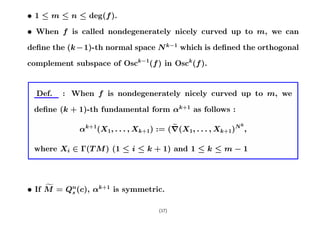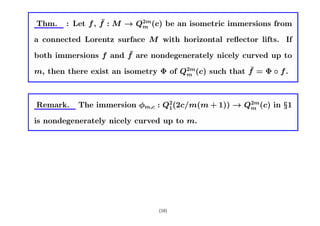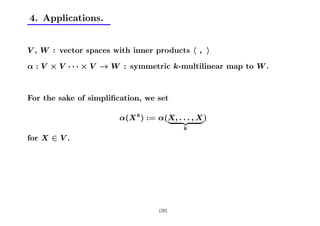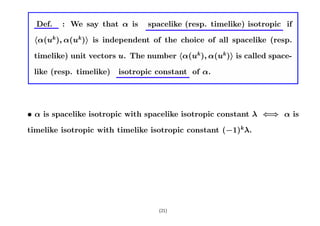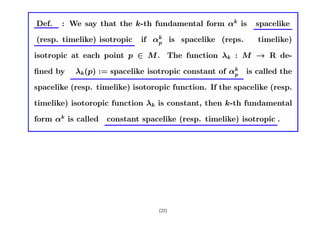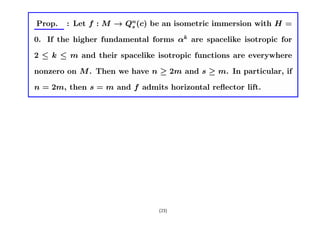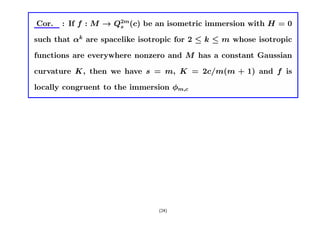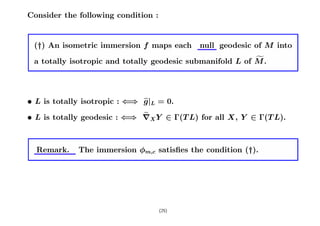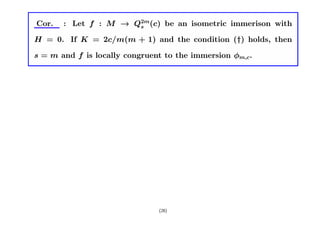1 von 26
Anzeige

### Reflect tsukuba524

1. Lorentz surfaces in pseudo-Riemannian space forms of neutral signature with horizontal reﬂector lifts Kazuyuki HASEGAWA (Kanazawa University) Joint work with Dr. K. Miura (Ochanomizu Univ.) 0. Introduction. f e (M , g ) : oriented n-dimensional pseudo-Riemannian manifold (M, g) : oriented pseudo-Riemannian submanifold f f : M → M : isometric immersion H : mean curvature vector ﬁeld (1)
2. Problem. f : Classify f : M → M with H = 0 • f Riemannian case : When M = S n(c), M = S 2(K) of constant curvatures c > 0 and K > 0, a full immersion f with H = 0 is congru- ent to the standard immersion (E. Calabi, J. Diﬀ. Geom., 1967). The f twistor space of M = S n(c) and the twistor lift play an important role. f • In this talk, we consider the problem above in the case of M = Qn(c) = s pseudo-Riemannian space form of constant curvature c and index s, and M =Lorentz surfaces , in particular, n = 2m and s = m. (2)
3. • This talk is consists of 1. Examples of Lorentz surfaces in pseudo-Riemannian space forms with H = 0. 2. Reﬂector spaces and reﬂector lifts. 3. A Rigidity theorem. 4. Applications. (3)
4. 1. Examples of Lorentz surfaces with H = 0. • In “K. Miura, Tsukuba J. math., 2007”, pseudo-Riemannian subman- ifolds of constant curvature in pseudo-Riemannian space forms with H = 0 are constructed from the Riemannian standard immersion us- ing “ Wick rotations ”. K[x] := K[x1, . . . , xn+1] : polynomial algebra in n + 1 variables x1, . . . , xn+1 over K (K = R or C). Kd[x] ⊂ K[x] : space of d-homogeneous polynomials Rn+1 : pseudo-Euclidean space of dim=n + 1 and index=t t (4)
5. n+1 X ⎪ ⎨ −1 ∂ (1 ≤ i ≤ t) 4Rn+1 := − εi , where εi = t ∂x2 i ⎪ ⎩ 1 (t + 1 ≤ i ≤ n + 1) i=1 H(Rn+1) := Ker4Rn+1 t t Hd(Rn+1) := H(Rn+1) ∩ Kd[x] t t (5)
6. We deﬁne ρt : C[x] → C[x] by ⎧ ⎪√ ⎨ −1xi (1 ≤ i ≤ t) ρt(xi) = ⎪ ⎩ xi (t + 1 ≤ i ≤ n + 1) • ρt is called “Wick rotation”. Set σt := ρt ◦ ρt and Pt± := Ker(σt|R[x] ∓ idR[x]). We deﬁne H± (Rn+1) := Pt± ∩ Hd(Rn+1). d,t 0 0 (6)
7. • Hd(Rn+1) = H+ (Rn+1) ⊕ H− (Rn+1) 0 d,t 0 d,t 0 {ui}i :orthonormal basis Hd(Rn+1) s.t. 0 u1, . . . , ul ∈ H− (Rn+1), d,t 0 ul+1, . . . um+1 ∈ H+ (Rn+1), d,t 0 m+1 X (ui)2 = (x2 + · · · + x2 )2, 1 n+1 i=1 where l = l(d, t) = dim H− (Rn+1) and m+1 = m(d, t)+1 = dim Hd(Rn+1). d,t 0 0 (7)
8. Hereafter, we assume n = 2 and t = 1 (for simplicity). Then we see that m = 2d + 1 and l = d. We deﬁne Ui := Imρt(ui) (i = 1, . . . , d) and Ui := Reρt(ui) (i = d + 1, . . . , 2d + 1) d(d+1) Using these function, we deﬁne an immersion from φ : Q2(1) → Q2d( 1 d 2 ) by φ = (U1, . . . , U2d+1). Note that φ satisﬁes H = 0. (8)
9. For example, when d = 2 (n = 1, t = 1), φ : Q2(1) → Q4(3) is given by 1 2 √ 3 1 2 U1 = ( +2x + y + z ), U2 = (y − z 2) 2 2 2 6 2 U3 = xy, U4 = zx, U5 = yz, d(d+1) • Composing homotheties and anti-isometries of Q2(1) and Q2d( 1 d 2 ), we can obtain immersions from Q2(2c/d(d + 1)) to Q2d(c) with H = 0 1 d 6 (c = 0). We denote this immersion by φd,c . (9)
10. 2. Reﬂector spaces and reﬂector lifts. (See “G. Jensen and M. Rigoli, Matematiche (Catania) 45 (1990), 407- 443. ”) f e (M , g ) : oriented 2m-dim. pseudo-Riemannian manifold of neutral signature (index= 1 dim M ) f 2 f Jx ∈ End(TxM ) s.t. 2 ◦ Jx = I, ◦ (Jx)∗gx = −ex, e g ◦ dim Ker(Jx − I) = dim Ker(Jx + I) = m, ◦ Jx preserves the orientation. (10)
11. f Zx : the set of such Jx ∈ End(TxM ) [ f Z(M ) := Zx f x∈M (the bundle whose ﬁbers are consist of para-complex structures) f • Z(M ) is called the f reﬂector space of M , which is one of corre- sponding objects to the twistor space in Riemannian geometry. (M, g) : oriented Lorentz surface with para-complex structure J ∈ Z(M ). f f : M → M : isometric immersion (11)
12. e f e Def. : The map J : M → Z(M ) satisfying J (x)|TxM = f∗ ◦ Jx is reﬂector lift of f . e e e Def. : The reﬂector lift is called horizontal if ∇J = 0, where ∇ is f the Levi-Civita connection of M . e e • ∇J = 0 ⇒ H = 0 • Surfaces with horizontal reﬂector lifts are corresponding to superminimal surfaces in Riemannian cases. (12)
13. Prop. The immersion φm,c : Q2(2c/m(m + 1)) → Q2m(c) in §1 1 m admits horizontal reﬂector lift. (13)
14. 3. A Rigidity theorem. At ﬁrst, we recall the k-th fundamental forms αk . f e (M , g ) : oriented n-dim pseudo-Riemannian manifold (M, g) : oriented pseudo-Riemannian submanifold f f : M → M : isometric immersion e f ∇ : Levi-Civita connection of M . e e e We deﬁne ∇X1 := X1, ∇(X1, X2) = ∇X1 X2 and inductively for k ≥ 3 e e e ∇(X1, . . . , Xk ) = ∇X1 ∇(X2, . . . , Xk ), where Xi ∈ Γ(T M ). (14)
15. We deﬁne e Osck (f ) := {(∇(X1, . . . , Xk ))x | Xi ∈ Γ(T M ), 1 ≤ i ≤ k} x and [ k Osc (f ) := Osck (f ). x x∈M f • T M = Osc1(f ) ⊂ Osc2(f ) ⊂ · · · ⊂ Osck (f ) ⊂ · · · ⊂ T M . • We deﬁne deg(f ) =the minimum integer d such that Oscd−1(f ) ( Oscd(f ) and Oscd(f ) = Oscd+1(f ). (15)
16. • There exists the maximum number n (1 ≤ n ≤ deg(f )) such that f Osc1(f ), . . . , Oscn(f ) are subbundles on T M . Then we call f to be nicely curved up to order n. • When f is nicely curved up to order n, there exists the maximum number m (1 ≤ m ≤ n) such that all induced metrics of subbundles Osc1(f ), . . . , Oscm(f ) are nondegenerate. Then f is called nondegenerately nicely curved up to m. (16)
17. • 1 ≤ m ≤ n ≤ deg(f ). • When f is called nondegenerately nicely curved up to m, we can deﬁne the (k −1)-th normal space N k−1 which is deﬁned the orthogonal complement subspace of Osck−1(f ) in Osck (f ). Def. : When f is nondegenerately nicely curved up to m, we deﬁne (k + 1)-th fundamental form αk+1 as follows : k e αk+1(X1, . . . , Xk+1) := (∇(X1, . . . , Xk+1)N , where Xi ∈ Γ(T M ) (1 ≤ i ≤ k + 1) and 1 ≤ k ≤ m − 1 f • If M = Qn(c), αk+1 is symmetric. s (17)
18. Thm. ¯ : Let f , f : M → Q2m(c) be an isometric immersions from m a connected Lorentz surface M with horizontal reﬂector lifts. If ¯ both immersions f and f are nondegenerately nicely curved up to ¯ m, then there exist an isometry Φ of Q2m(c) such that f = Φ ◦ f . m Remark. The immersion φm,c : Q2(2c/m(m + 1)) → Q2m(c) in §1 1 m is nondegenerately nicely curved up to m. (18)
19. Cor. : Let f : M → Q2m(c) be an isometric immersions from a m connected Lorentz surface M with horizontal reﬂector lifts. If f is nondegenerately nicely curved up to m and the Gaussian curvature K is constant, then K = 2c/m(m + 1) and f is locally congruent to φm,c. (19)
20. 4. Applications. V , W : vector spaces with inner products h , i α : V × V · · · × V → W : symmetric k-multilinear map to W . For the sake of simpliﬁcation, we set α(X k ) := α(X, .{z , X ) | .. } k for X ∈ V . (20)
21. Def. : We say that α is spacelike (resp. timelike) isotropic if hα(uk ), α(uk )i is independent of the choice of all spacelike (resp. timelike) unit vectors u. The number hα(uk ), α(uk )i is called space- like (resp. timelike) isotropic constant of α. • α is spacelike isotropic with spacelike isotropic constant λ ⇐⇒ α is timelike isotropic with timelike isotropic constant (−1)k λ. (21)
22. Def. : We say that the k-th fundamental form αk is spacelike (resp. timelike) isotropic if αk is spacelike (reps. p timelike) isotropic at each point p ∈ M . The function λk : M → R de- ﬁned by λk (p) := spacelike isotropic constant of αk p is called the spacelike (resp. timelike) isotoropic function. If the spacelike (resp. timelike) isotoropic function λk is constant, then k-th fundamental form αk is called constant spacelike (resp. timelike) isotropic . (22)
23. Prop. : Let f : M → Qn(c) be an isometric immersion with H = s 0. If the higher fundamental forms αk are spacelike isotropic for 2 ≤ k ≤ m and their spacelike isotropic functions are everywhere nonzero on M . Then we have n ≥ 2m and s ≥ m. In particular, if n = 2m, then s = m and f admits horizontal reﬂector lift. (23)
24. Cor. : If f : M → Q2m(c) be an isometric immersion with H = 0 s such that αk are spacelike isotropic for 2 ≤ k ≤ m whose isotropic functions are everywhere nonzero and M has a constant Gaussian curvature K, then we have s = m, K = 2c/m(m + 1) and f is locally congruent to the immersion φm,c (24)
25. Consider the following condition : (†) An isometric immersion f maps each null geodesic of M into f a totally isotropic and totally geodesic submanifold L of M . e • L is totally isotropic : ⇐⇒ g |L = 0. e • L is totally geodesic : ⇐⇒ ∇X Y ∈ Γ(T L) for all X, Y ∈ Γ(T L). Remark. The immersion φm,c satisﬁes the condition (†). (25)
26. Cor. : Let f : M → Q2m(c) be an isometric immerison with s H = 0. If K = 2c/m(m + 1) and the condition (†) holds, then s = m and f is locally congruent to the immersion φm,c. (26)
Anzeige